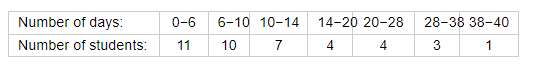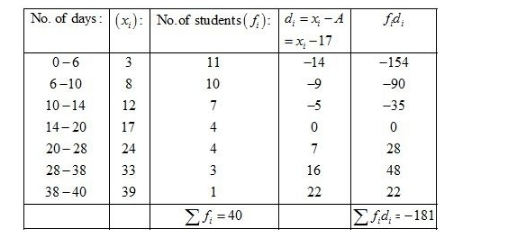# A class teacher has the following absentee record of 40 students of a class for the whole term.

Question:

A class teacher has the following absentee record of 40 students of a class for the whole term. Find the mean number of days a student was absent.Solution:

Let the assume mean = 17.We know that mean, $\bar{X}=A+\frac{1}{N} \sum_{i=1}^{n} f_{i} d_{i}$

Now, we have $N=\sum f_{i}=40, \sum f_{i} d_{i}=181$ and $A=17$.

Putting the values in the above formula, we have

$\bar{X}=A+\frac{1}{N} \sum_{i=1}^{n} f_{i} d_{i}$

$=17+\frac{1}{40} \times(-181)$

$=17-\frac{181}{40}$

$=17-4.525$

$=12.475$

Hence, the mean number of days a student was absent is 12.475.